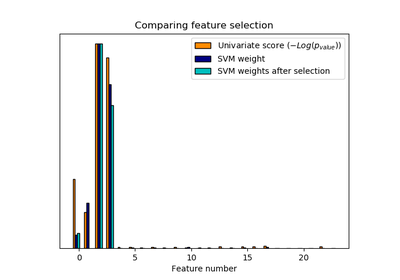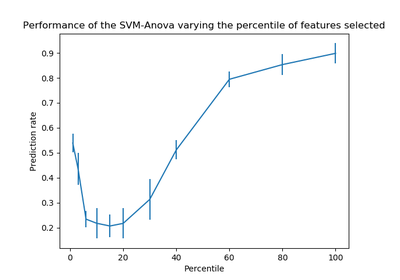# `sklearn.feature_selection`.f_classif¶

`sklearn.feature_selection.``f_classif`(X, y)[source]

Compute the ANOVA F-value for the provided sample.

Read more in the User Guide.

Parameters: X : {array-like, sparse matrix} shape = [n_samples, n_features] The set of regressors that will be tested sequentially. y : array of shape(n_samples) The data matrix. F : array, shape = [n_features,] The set of F values. pval : array, shape = [n_features,] The set of p-values.

`chi2`
Chi-squared stats of non-negative features for classification tasks.
`f_regression`
F-value between label/feature for regression tasks.

## Examples using `sklearn.feature_selection.f_classif`¶Univariate Feature SelectionSVM-Anova: SVM with univariate feature selection